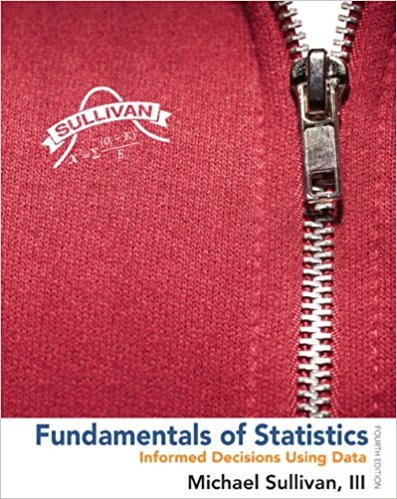×
×

# Use a normal probability plot to assess whether the sampleISBN: 9780321838704 30

## Solution for problem 11AYU Chapter Chapter 7.3

Fundamentals of Statistics | 4th Edition

• Textbook Solutions
• 2901 Step-by-step solutions solved by professors and subject experts
• Get 24/7 help from StudySoup virtual teaching assistantsFundamentals of Statistics | 4th Edition

4 5 1 336 Reviews
23
3
Problem 11AYU

Use a normal probability plot to assess whether the sample data could have come from a population that is normally distributed. O-Ring Thickness A random sample of O-rings was obtained, and the wall thickness (in inches) of each was recorded.

 0.276 0.274 0.275 0.274 0.277 0.273 0.276 0.276 0.279 0.274 0.273 0.277 0.275 0.277 0.277 0.276 0.277 0.278 0.275 0.276
Step-by-Step Solution:

Step 1 :

From the Excel

> arrange x - values in ascending order

> calculate, i is the position of the data

> find z score for each> plot x - values on the horizontal axis and the corresponding z-score on the vertical axis

...
 x position=Z - value 0.273 1 0.030864 -1.86824 0.273 2 0.080247 -1.40341 0.274 3 0.12963 -1.12814 0.274 4 0.179012 -0.91914 0.274 5 0.228395 -0.74414 0.275 6
Step 2 of 3

Step 3 of 3

##### ISBN: 9780321838704

Unlock Textbook Solution